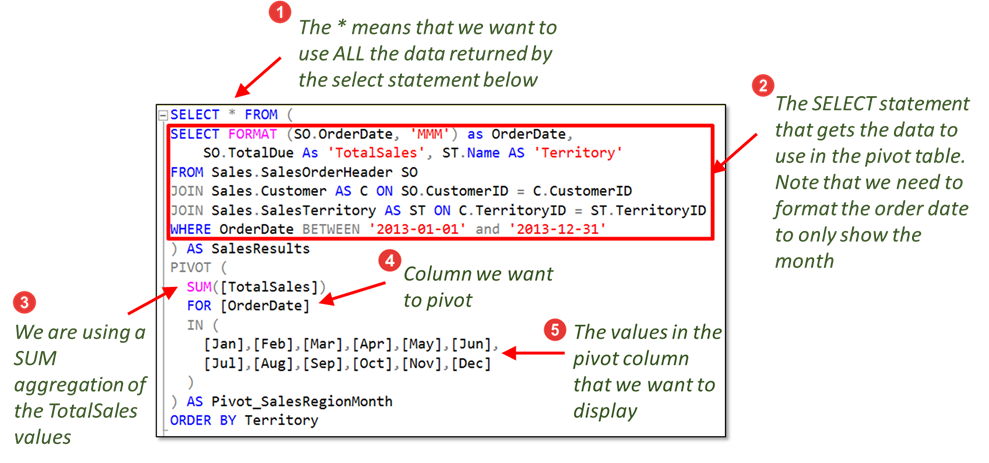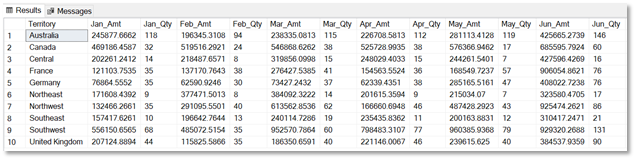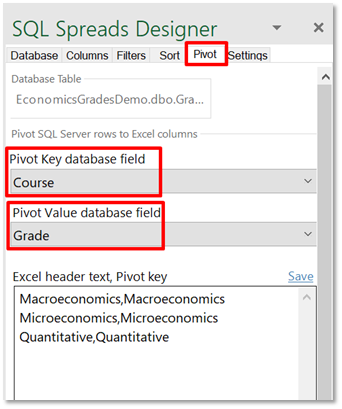• # How to create Pivot Tables in SQL Server

Oct 10th, 2021

In this article, we’re going to show you how to create pivot tables in SQL Server.  This is the third article aimed at teaching Excel users some basic SQL server queries, and how to mimic some of the main functions used in Excel. In a previous article we looked at the equivalent of Excel’s VLOOKUP in SQL, and now we’re going to look at an equally popular Excel feature, the Pivot Table.

## Pivot Tables in Excel

If you use Excel often, then you’ll be very familiar with pivot tables and understand their importance.  Why are they so important?  Put simply, they allow you to extract information quickly and easily from large quantities of data.  Or to think of it another way, if you need to answer questions about your data, then a pivot table is usually the best place to start.  Let’s look at an example to illustrate this.

The table below shows sales data for a company.  There’s a lot of data in this table, so to start analyzing it and trying to find information on how sales are doing, we’re going to need to do some initial aggregations – using pivot tables!The first couple of questions we might ask to find out more about the company sales performance are:

• How have sales changed over time?
• Do certain regions have higher sales than others?
• Which categories have higher sales?

We could answer these very easily by creating two simple pivot tables.We can see straight away overall, sales have generally been increasing, although sales for the South region dropped in 2015 and 2016.  Also, we can also see that across all regions, Office Supplies and Technology were down in 2015.  This is a great start, and we can drill in further with additional pivot tables. All this is done very quickly and easily with the pivot table feature in Excel.

The bottom line is that pivot tables are very powerful and can be used to analyze large data sets in the following ways:

• Produce aggregated summaries (eg sum, count, average)
• Identify trends and patterns
• Produce simple and concise reports

So how would we create similar pivot tables in SQL Server?  Microsoft introduced the PIVOT operator was introduced in SQL Server 2005, and it is this approach that we’re going to discuss.

## PIVOT tables in SQL Server

We’re going to use the AdventureWorks sample database to create our PIVOT example.  You can get the database file and installation instructions here. The following query produces a list of sales results which we want to summarize in a pivot table report to show ‘Sales per month by region’.

Note that we need to retrieve the data that we need from several tables in the AdventureWorks database.  We do this using the JOIN clause.  If you are not familiar with joining tables, then check out one of our previous articles here.

``````SELECT SO.CustomerID, SO.SalesOrderNumber, SO.OrderDate, SO.TotalDue, ST.Name AS 'Territory'
JOIN Sales.Customer AS C ON SO.CustomerID = C.CustomerID
JOIN Sales.SalesTerritory AS ST ON C.TerritoryID = ST.TerritoryID
WHERE OrderDate BETWEEN '2013-01-01' and '2013-12-31';``````First, let’s copy the results from SQL Server Management Studio and paste them into Excel so that we can create the pivot table that we’re going to re-produce in SQL.### PIVOT operator syntax

The PIVOT operator has the following structure:

``````SELECT
<the data you want to display>
FROM
(
<the SELECT statement that gets the data>
) AS <Alias for temporary table>
PIVOT
(
<aggregation function>(<column being aggregated>)
FOR [<column that contains the values that will become column headers>]
IN ( [first pivoted column], [second pivoted column], ...
[last pivoted column])
) AS <alias for the pivot table>
<optional ORDER BY clause>;``````

As you can see, the script has two main parts: the first part gets the data, and the second part defines the structure of the pivot table.  If we look at the second part in more detail, there are three keywords we need to understand.

• The AGGREGATION function specifies how to aggregate the values from the select query. We can use SUM, COUNT, AVG, MIN or MAX, depending on what we want in the pivot table
• The FOR keyword tells the PIVOT operator which column will be pivoted, ie the column that contains the values that will become column headers in the pivot table
• The IN keyword lists all the distinct values from the column we are pivoting and that we want to see as column headers

The syntax becomes much easier to understand with real data, so let’s go back to our AdventureWorks example.

### The PIVOT Operator in action

The script below uses the example select query that we looked at earlier to get sales data for 2013 and display it per region by month.

``````SELECT * FROM (
SELECT FORMAT (SO.OrderDate, 'MMM') as OrderDate, SO.TotalDue As 'TotalSales', ST.Name AS 'Territory'
JOIN Sales.Customer AS C ON SO.CustomerID = C.CustomerID
JOIN Sales.SalesTerritory AS ST ON C.TerritoryID = ST.TerritoryID
WHERE OrderDate BETWEEN '2013-01-01' and '2013-12-31'
) AS SalesResults
PIVOT (
SUM([TotalSales])
FOR [OrderDate]
IN (
[Jan],[Feb],[Mar],[Apr],[May],[Jun],[Jul],[Aug],[Sep],[Oct],[Nov],[Dec]
)
) AS Pivot_SalesRegionMonth
ORDER BY Territory;``````Let’s break down the script a little more to clarify the syntax.1. The * in the first SELECT statement means that we want to use all the columns returned by the SELECT statement below that actually gets the data. We could also explicitly state the columns by using ‘SELECT Territory,[Jan],[Feb],[Mar],[Apr],[May],[Jun],[Jul],[Aug],[Sep],[Oct],[Nov],[Dec] FROM…’, but using * is easier.
2. In the main SELECT statement, note that we only specify the columns that will be used in the pivot table: the OrderDate (which is our pivoted column), the ‘Territory’ (which are our aggregated row headings), and the ‘TotalSales’ (which are the values we are aggregating). Note also that we need to effectively ‘group’ the sales order dates into months using the FORMAT function – Excel would typically automatically group dates for you in pivot tables.
3. The aggregation function in our example uses SUM
4. The FOR keyword specifies that we want to pivot the OrderDate column
5. The IN keyword lists the values from the OrderDate column that we want to see displayed as column headers in our pivot table report. Note that we need to ‘hardcode’ these into our query – in our example, we have therefore listed all months from Jan to Dec.

But wait!  The results from our query don’t include a ‘Grand Total’ column which is included by default when you create a pivot table in Excel.To include a total column, we need to add an additional line to our script.## PIVOT Operator – limitations

For those Excel users that are used to the pivot table feature in Excel, then you’ll notice some differences with the PIVOT operator in SQL.  We could refer to them as ‘limitations’ because in Excel the pivot table feature has no such problems.

The first is that the PIVOT operator can include only one aggregation.  For example, if we wanted to see the number of sales orders in each month for each region alongside the sum, then we can’t just add COUNT(TotalSales) to our script.

The other main limitation is that the IN clause of the PIVOT operator accepts only a hard-coded list of distinct values from the column we are pivoting and that we want to see as column headers.  In other words, we need to know what our pivot column headers are going to be before we write the query.  This is ok in our example as we know that there are 12 months in the year.  However, in most other cases this is a problem, as it means we need to edit the script every time a new pivot needs to be added.

There are workarounds to both limitations, which we’ll look at now.

### Multi aggregate Pivot

#### Using multiple PIVOT operators

The PIVOT operator can include only one aggregation.  To include multiple aggregations with the PIVOT operator we would need to have multiple pivots and join them, as well as adding all the necessary columns to the Select list.

Here is how we could use two pivots to return the total amount and total quantity for each month in each region.

``````  SELECT amt.Territory,
amt.[Jan] As 'Jan_Amt',
qty.[Jan] As 'Jan_Qty',
amt.[Feb] As 'Feb_Amt',
qty.[Feb] As 'Feb_Qty',
amt.[Mar] As 'Mar_Amt',
qty.[Mar] As 'Mar_Qty',
amt.[Apr] As 'Apr_Amt',
qty.[Apr] As 'Apr_Qty',
amt.[May] As 'May_Amt',
qty.[May] As 'May_Qty',
amt.[Jun] As 'Jun_Amt',
qty.[Jun] As 'Jun_Qty',
amt.[Jul] As 'Jul_Amt',
qty.[Jul] As 'Jul_Qty',
amt.[Aug] As 'Aug_Amt',
qty.[Aug] As 'Aug_Qty',
amt.[Sep] As 'Sep_Amt',
qty.[Sep] As 'Sep_Qty',
amt.[Oct] As 'Oct_Amt',
qty.[Oct] As 'Oct_Qty',
amt.[Nov] As 'Nov_Amt',
qty.[Nov] As 'Nov_Qty',
amt.[Dec] As 'Dec_Amt',
qty.[Dec] As 'Dec_Qty'
FROM
(SELECT FORMAT (SO.OrderDate, 'MMM') as 'OrderDate',
SO.TotalDue As 'TotalSales', ST.Name AS 'Territory'
JOIN Sales.Customer AS C ON SO.CustomerID = C.CustomerID
JOIN Sales.SalesTerritory AS ST ON C.TerritoryID = ST.TerritoryID
WHERE OrderDate BETWEEN '2013-01-01' and '2013-12-31'
) AS SalesTotal
PIVOT (SUM(TotalSales) FOR [OrderDate] IN ([Jan],[Feb],[Mar],[Apr],[May],[Jun],[Jul],[Aug],[Sep],[Oct],[Nov],[Dec])) AS amt
INNER JOIN
(SELECT FORMAT (SO.OrderDate, 'MMM') as 'OrderDate',
SO.TotalDue As 'TotalSales', ST.Name AS 'Territory'
JOIN Sales.Customer AS C ON SO.CustomerID = C.CustomerID
JOIN Sales.SalesTerritory AS ST ON C.TerritoryID = ST.TerritoryID
WHERE OrderDate BETWEEN '2013-01-01' and '2013-12-31'
) AS SalesCount
PIVOT (COUNT(TotalSales) FOR [OrderDate] IN ([Jan],[Feb],[Mar],[Apr],[May],[Jun],[Jul],[Aug],[Sep],[Oct],[Nov],[Dec])) AS qty
ON qty.Territory = amt.Territory
ORDER BY amt.Territory;``````

Here is what the results look like.As you can see, it’s quite an effort to get the results that we want using multiple PIVOT operators.  There is, however, another way to do this which doesn’t use the PIVOT operator at all.

#### Using CASE and GROUP BY statements

The CASE statement acts as a logical IF…THEN…ELSE expression and returns various values depending on the result.  We can wrap it in a SUM aggregation and a COUNT aggregation to get the totals we need per month and the GROUP BY statement to display by Territory.

Here is the query:

``````SELECT     ST.Name AS 'Territory',
SUM(CASE WHEN MONTH(SO.OrderDate) = 1 THEN SO.TotalDue ELSE 0 END) AS 'Jan_Amt',
COUNT(CASE WHEN MONTH(SO.OrderDate) = 1 THEN SO.TotalDue ELSE NULL END) AS 'Jan_Qty',
SUM(CASE WHEN MONTH(SO.OrderDate) = 2 THEN SO.TotalDue ELSE 0 END) AS 'Feb_Amt',
COUNT(CASE WHEN MONTH(SO.OrderDate) = 2 THEN SO.TotalDue ELSE NULL END) AS 'Feb_Qty',
SUM(CASE WHEN MONTH(SO.OrderDate) = 3 THEN SO.TotalDue ELSE 0 END) AS 'Mar_Amt',
COUNT(CASE WHEN MONTH(SO.OrderDate) = 3 THEN SO.TotalDue ELSE NULL END) AS 'Mar_Qty',
SUM(CASE WHEN MONTH(SO.OrderDate) = 4 THEN SO.TotalDue ELSE 0 END) AS 'Apr_Amt',
COUNT(CASE WHEN MONTH(SO.OrderDate) = 4 THEN SO.TotalDue ELSE NULL END) AS 'Apr_Qty',
SUM(CASE WHEN MONTH(SO.OrderDate) = 5 THEN SO.TotalDue ELSE 0 END) AS 'May_Amt',
COUNT(CASE WHEN MONTH(SO.OrderDate) = 5 THEN SO.TotalDue ELSE NULL END) AS 'May_Qty',
SUM(CASE WHEN MONTH(SO.OrderDate) = 6 THEN SO.TotalDue ELSE 0 END) AS 'Jun_Amt',
COUNT(CASE WHEN MONTH(SO.OrderDate) = 6 THEN SO.TotalDue ELSE NULL END) AS 'Jun_Qty',
SUM(CASE WHEN MONTH(SO.OrderDate) = 7 THEN SO.TotalDue ELSE 0 END) AS 'Jul_Amt',
COUNT(CASE WHEN MONTH(SO.OrderDate) = 7 THEN SO.TotalDue ELSE NULL END) AS 'Jul_Qty',
SUM(CASE WHEN MONTH(SO.OrderDate) = 8 THEN SO.TotalDue ELSE 0 END) AS 'Aug_Amt',
COUNT(CASE WHEN MONTH(SO.OrderDate) = 8 THEN SO.TotalDue ELSE NULL END) AS 'Aug_Qty',
SUM(CASE WHEN MONTH(SO.OrderDate) = 9 THEN SO.TotalDue ELSE 0 END) AS 'Sep_Amt',
COUNT(CASE WHEN MONTH(SO.OrderDate) = 9 THEN SO.TotalDue ELSE NULL END) AS 'Sep_Qty',
SUM(CASE WHEN MONTH(SO.OrderDate) = 10 THEN SO.TotalDue ELSE 0 END) AS 'Oct_Amt',
COUNT(CASE WHEN MONTH(SO.OrderDate) = 10 THEN SO.TotalDue ELSE NULL END) AS 'Oct_Qty',
SUM(CASE WHEN MONTH(SO.OrderDate) = 11 THEN SO.TotalDue ELSE 0 END) AS 'Nov_Amt',
COUNT(CASE WHEN MONTH(SO.OrderDate) = 11 THEN SO.TotalDue ELSE NULL END) AS 'Nov_Qty',
SUM(CASE WHEN MONTH(SO.OrderDate) = 12 THEN SO.TotalDue ELSE 0 END) AS 'Dec_Amt',
COUNT(CASE WHEN MONTH(SO.OrderDate) = 12 THEN SO.TotalDue ELSE NULL END) AS 'Dec_Qty'
JOIN Sales.Customer AS C ON SO.CustomerID = C.CustomerID
JOIN Sales.SalesTerritory AS ST ON C.TerritoryID = ST.TerritoryID
WHERE OrderDate BETWEEN '2013-01-01' and '2013-12-31'
GROUP BY ST.Name
ORDER BY ST.Name;``````

Here are the results.Although the query is still quite long, the structure of it is simpler to understand (at least to my eyes).  It also highlights how useful the CASE statement is – we’ll look more at this handy function in a later post.

### Dynamic Pivot

The IN clause of the PIVOT operator accepts only a hard-coded, comma-separated list of spreading element values.  In situations when the values are not known, we can use dynamic SQL to construct the query. We’re not going to go into the details here in this article because it introduces some more advanced SQL concepts.  There is a great explanation of how to create a dynamic pivot here.

## Pivoting Data between Excel and SQL Server with SQL Spreads

We’ve seen how we can create pivot tables in SQL Server to view data in much the same way as we’d use pivot tables in Excel.  What if we want to interact with data in SQL Server (ie update values) from a pivot table in Excel?  A use case for this kind of action would be an admin clerk updating monthly daily product sales figures, or a teacher filling in student grades.  In both cases, it’s much easier to perform data entry when the data is pivoted rather than working in a flat view.

Let’s take a look at the student grade use case with a simple example.  The screenshot below shows student results in a SQL Server database.First, we need to get the data into Excel from SQL Server by simply clicking on the relevant table in the SQL Spreads Designer.Now comes the clever part!  We’re going to pivot the table on the Course column, and in so doing, ‘placeholders’ will be created for all the data we need to fill in.  We do this by clicking on the Pivot tab in the SQL Spreads Designer and then specifying the column we want to pivot and the column which has the values in it.The data in Excel is now pivoted.  As you can see, there are a series of NULL’s to indicate where there is currently no grade value for a particular student/grade combination.We can now go ahead and enter the missing grades and click on ‘Save to Database’.  The updated results are now saved safely in SQL Server.## Summary

Pivot tables are one of the best and most useful features in Excel.  In this article, we’ve shown you how to create pivot tables in SQL Server.  We did this using the PIVOT operator in our SQL script.  However, we soon noticed a couple of limitations, which can be overcome through some additions to the SQL scripts which adds to the complexity.

The main takeaway should be that although you can create pivot tables in SQL, it is far easier to grab the results from SQL paste them into Excel where you can create better pivot tables in less time.  This is a great example of using Excel and SQL together to produce the best results – a philosophy we summarized in an earlier post: “SQL and Excel: Why you need both”.

Finally, we showed you how to use the SQL Spreads Excel Add-in to pivot data between Excel and SQL Server and make it much easier to perform updates on flat data in SQL.

To find out how to use SQL Spreads to make working with Excel and SQL even easier, download the trial today.